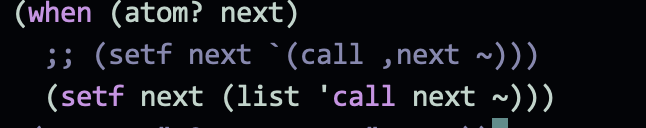# 求教: SBCL 的bug??

`(vprint (list (second next) '~) `(,(second next) ~))`

`(setf next `(,(second next) ~)))`

`(setf next (list (second next) '~))`http://clhs.lisp.se/Body/02_df.htm

An implementation is free to interpret a backquoted form F1 as any form F2 that, when evaluated, will produce a result that is the same under equal as the result implied by the above definition, provided that the side-effect behavior of the substitute form F2 is also consistent with the description given above. The constructed copy of the template might or might not share list structure with the template itself. As an example, the above definition implies that

`((,a b) ,c ,@d)

will be interpreted as if it were

(append (list (append (list a) (list 'b) ’ nil )) (list c) d ’ nil )

but it could also be legitimately interpreted to mean any of the following:

(append (list (append (list a) (list 'b))) (list c) d) (append (list (append (list a) '(b))) (list c) d) (list* (cons a '(b)) c d) (list* (cons a (list 'b)) c d) (append (list (cons a '(b))) (list c) d) (list* (cons a '(b)) c (copy-list d))

SBCL 定义了一个 compiler macro 把 backquote form 常量化了

``````* (describe 'SB-INT:QUASIQUOTE)
SB-INT:QUASIQUOTE
[symbol]

QUASIQUOTE names a macro:
Lambda-list: (SB-IMPL::THING)
Source file: SYS:SRC;CODE;BACKQ.LISP

QUASIQUOTE has a compiler-macro:
Source file: SYS:SRC;CODE;BACKQ.LISP
* (macroexpand '`(,(second next) ~) )
(LIST* (SECOND NEXT) '(~))
T
* (sb-impl::expand-quasiquote '`(,(second next) ~) t)
(SB-IMPL::|List| 'SB-INT:QUASIQUOTE
(SB-IMPL::|List*| (SB-INT:UNQUOTE '(SECOND NEXT)) '(~)))
* (setf *PRINT-PRETTY* nil)
NIL
* '`(,(second next) ~)
(SB-INT:QUASIQUOTE (#S(SB-IMPL::COMMA :EXPR (SECOND NEXT) :KIND 0) ~))
``````

``````(defconstant ~ '~)
``````

``````* (defvar *a* 12)
*A*
* (defun foo () `(,(+ *a* 2) ~))
FOO
* (foo)
(14 ~)
* (setq *a* 15)
15
* (foo)
(17 ~)
* (defparameter tmp (cons 11 (foo)))
(11 17 ~)
* tmp
(11 17 ~)
~
12
* (foo)
(17 12)
``````

``````* (defun foo2 () (list (+ *a* 2) '~))
FOO2
* (foo2)
(17 ~)
* (setq tmp (cons 12 (foo2)))
(12 17 ~)
1
* tmp
(12 17 1)
* (foo2)
(17 ~)
``````
1赞

a behavior or aspect of Common Lisp which has been deliberately left unspecified

1赞

ub = Unspecified Behavior
(未声明的行为，意味着实现者可以按照自己的方式实现以达到更佳效果）